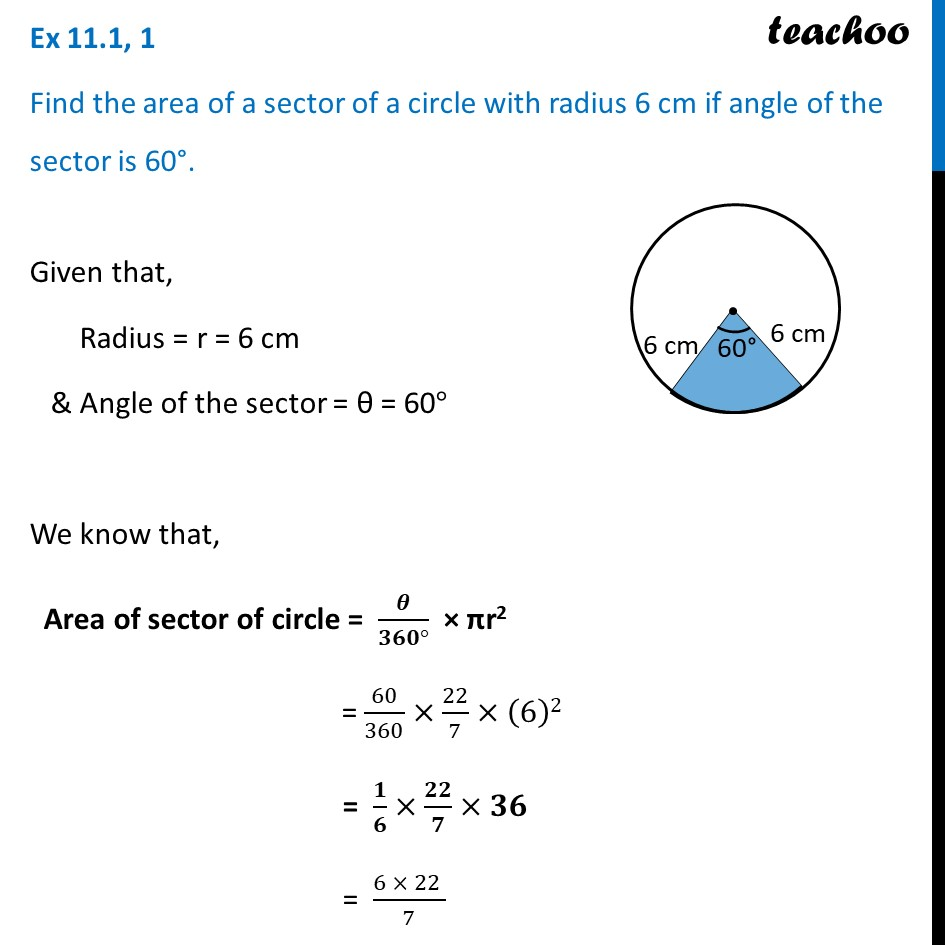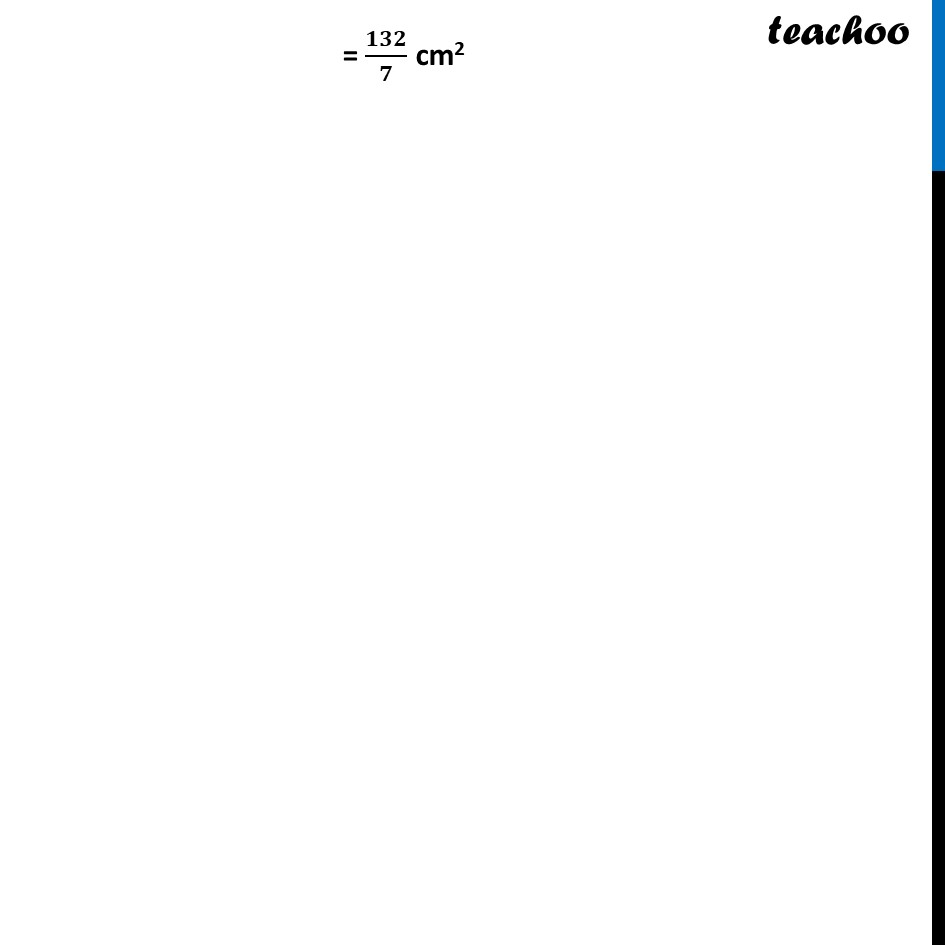Ex 11.1

Chapter 11 Class 10 Areas related to Circles
Serial order wiseLearn in your speed, with individual attention - Teachoo Maths 1-on-1 Class

### Transcript

Ex 11.1, 1 Find the area of a sector of a circle with radius 6 cm if angle of the sector is 60°. Given that, Radius = r = 6 cm & Angle of the sector = θ = 60° We know that, Area of sector of circle = 𝜽/(𝟑𝟔𝟎°) × πr2 = 60/360×22/7×(6)2 = 𝟏/𝟔×𝟐𝟐/𝟕×𝟑𝟔 = (6 × 22 )/7 = 132/7 cm2